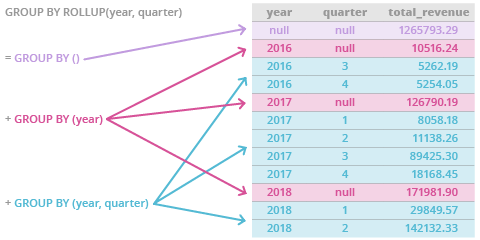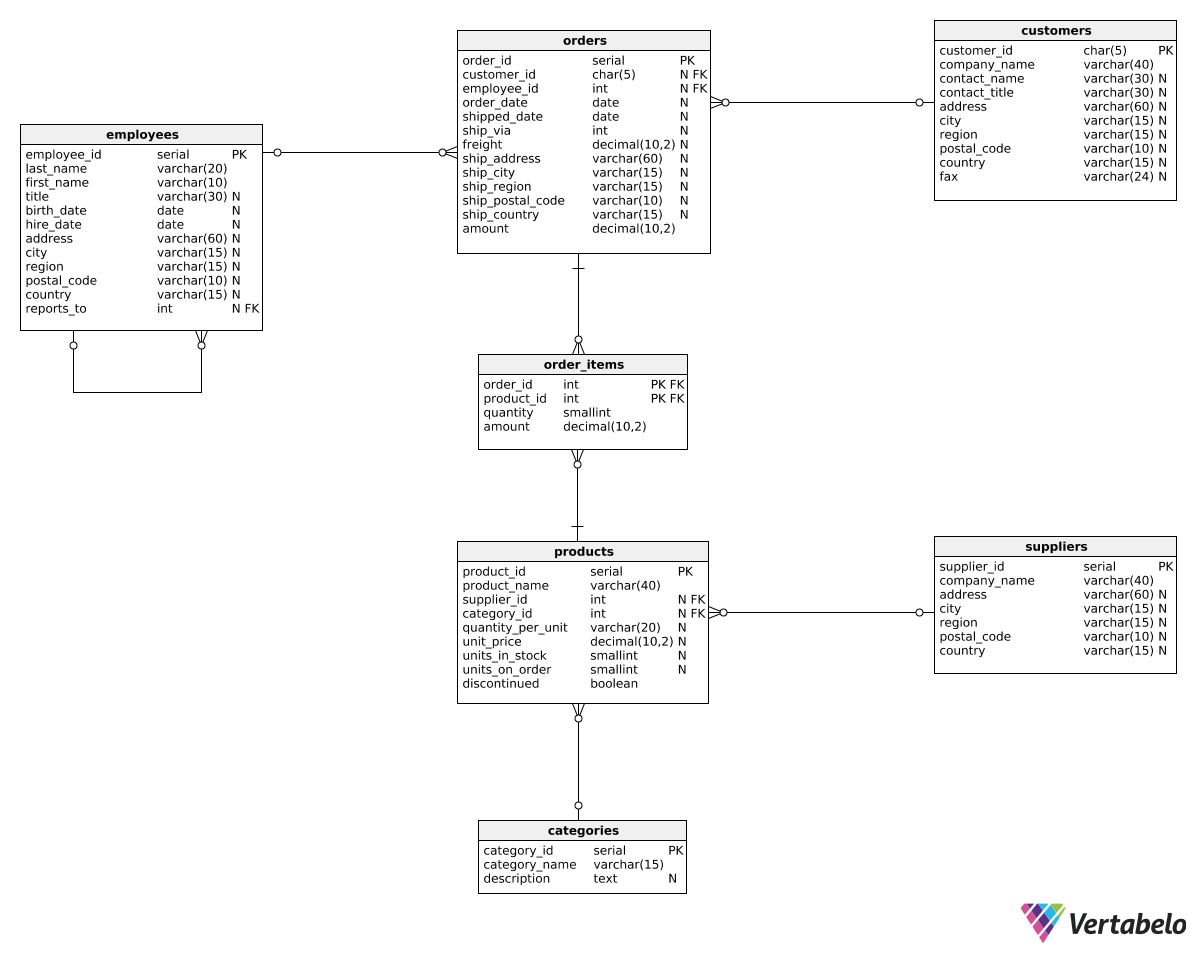Deals Of The Week - hours only!Up to 80% off on all courses and bundles.-Close
Introduction
Total revenue for multiple periods
Calculating deltas
Creating "revenue in quarters" reports
Summary

## Instruction

Excellent! As you saw, we used ROLLUP() in the GROUP BY clause:

SELECT
EXTRACT(year FROM order_date) AS revenue_year,
EXTRACT(quarter FROM order_date) AS revenue_quarter,
SUM(amount) AS total_revenue
FROM orders
GROUP BY ROLLUP(
EXTRACT(year FROM order_date),
EXTRACT(quarter FROM order_date)
)
ORDER BY
EXTRACT(year FROM order_date),
EXTRACT(quarter FROM order_date);


In SQL Server, ROLLUP() is an extension of GROUP BY. Inside the brackets, we provide all the columns that we want to group the rows by. ROLLUP() first groups the rows by both columns (in this case, year and quarter) to compute quarterly sums. Then, it groups all rows by the first column inside the parentheses (year) to compute the annual sums. Finally, it computes a grand total, i.e. as if we didn't group the rows by anything.

As a general rule, ROLLUP() always creates new grouping combinations by removing columns one by one, starting from the right:

GROUP BY ROLLUP (A, B, C) =
GROUP BY (A, B, C) +
GROUP BY (A, B) +
GROUP BY (A) +
GROUP BY ()


The diagram below explains where the resulting rows come from:In this course, we're only going to briefly introduce ROLLUP(). To learn more about this and other GROUP BY extensions, check out our GROUP BY Extensions in SQL course.

## Exercise

Show the total revenue generated by all orders on three aggregation levels:

1. The grand total of all revenue values – total_revenue
2. Annual revenue values – revenue_year
3. Monthly revenue values – revenue_month

Order all rows by year and month.

### Stuck? Here's a hint!

Use the following GROUP BY clause:

GROUP BY ROLLUP(
EXTRACT(year FROM order_date),
EXTRACT(month FROM order_date)
)